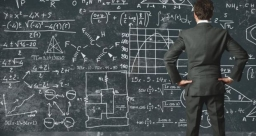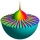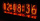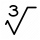# Fraction + eq

Solve following simple equation with fractions: -5/6(8+5b) = 75 + 5/3b

b =  -14

### Step-by-step explanation:

-5/6(8+5b) = 75 + 5/3b

-5/6•(8+5•b) = 75 + 5/3•b

35b = -490

b = -14

Our simple equation calculator calculates it.Did you find an error or inaccuracy? Feel free to write us. Thank you!Math student
2/6 divided by 2/4Dr MathTips to related online calculators
Need help to calculate sum, simplify or multiply fractions? Try our fraction calculator.
Do you have a linear equation or system of equations and looking for its solution? Or do you have a quadratic equation?

## Related math problems and questions:

• Sum of fractionsWhat is the sum of 2/3+3/5?
• Mixed numbersRewrite mixed numbers, so the fractions have the same denominator: 5 1/5 - 2 2/3
• Watching TVOne evening 2/3 students watch TV. Of those students, 3/8 watched a reality show. Of the students that watched the show, 1/4 of them recorded it. What fraction of the students watched and recorded reality tv.
• Linear combination of complexIf z1=5+3i and z2=4-2i, write the following in the form a+bi a) 4z1+6z2 b) z1*z2
• Simplify 3Simplify mixed numerals expression: 8 1/4- 3 2/5 - (2 1/3 - 1/4) Show your solution.
• Turtles 2A box turtle hibernates in the sand at 11 5/8. A spotted turtle hibernates at 11 16/25 feet. Which turtle is deeper? Write answer as number 1 or 2.
• Evaluate 5Evaluate expression x2−7x+12x−4 when x=−1The heights of five starters on redwood high’s basketball team are 5’11”, 6’3”, 6’6”, 6’2” and 6’. The average of height of these players is?
• LCD two fractionsWhat is the least common denominator of 11/15 and 12/19?
• BoatsThree-quarters of boats are white, 1/7 are blue and 9 are red. How many boats do we have?
• Std-deviationCalculate standard deviation for file: 63,65,68,69,69,72,75,76,77,79,79,80,82,83,84,88,90
• Eq2 2Solve following equation with quadratic members and rational function: (x2+1)/(x-4) + (x2-1)/(x+3) = 23
• 75th percentile (quartille Q3)Find 75th percentile for 30,42,42,46,46,46,50,50,54
• Solve 2Solve integer equation: a +b+c =30 a, b, c = can be odd natural number from this set (1,3,5,7,9,11,13,15)
• Standard deviationFind standard deviation for dataset (grouped data): Age (years) No. Of Persons 0-10 15 10-20 15 20-30 23 30-40 22 40-50 25 50-60 10 60-70 5 70-80 10
• EmilyEmily had 20 minutes to do a three-problem quiz. She spent 11 3/4 minutes on question A and 5 1/2 minutes on question B. How much time did she have left for question C?
• One third powerWhich equation justifies why ten to the one-third power equals the cube root of ten?